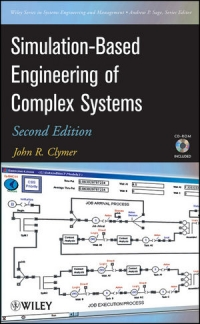## Simulation-Based Engineering of Complex Systems (Wiley Series in Systems Engineering and Management)

Clymer, John R.
WileyBlackwell: Hoboken, NJ, 2009
ISBN 9780470401293 (pb)

Order this book

Reviewed by Ipek Bozkurt
Engineering Management Program, University of Houston-Clear LakeComplex systems have been of interest to researchers in various disciplines, and despite the body of knowledge accumulated as of today, different ways of dealing with them are still being sought. Readers who are not fully familiar with the vast amount of research on "complex systems" and "complex adaptive systems" could benefit from gaining information on these concepts prior to reading this book. The main goal of this book is to shed light on complex systems through simulation-based engineering. The book would be difficult to understand and follow for undergraduate-level students. However, once the necessary basic knowledge of systems engineering and modeling and simulation is obtained, the book may be used as both a textbook for graduate students, and also a reference book for practitioners, as the author notes.

Chapter 1 is intended to provide an introduction to simulation-based systems engineering. Starting with what complex systems are, the author follows with how simulation can be used to understand complex systems. Following a very brief introduction to Operational Evaluation Modeling (OpEM) and Operational Evaluation Modeling of Context-Sensitive Systems (OpEMCSS), two example models are discussed for the purpose of showing how OpEMCSS can be used to achieve understanding of complex systems.

Chapter 2 begins with a brief summary of the statistical aspects of simulation, followed by a description of Petri Nets. The definition and discussion of OpEM graphs and comparison of the OpEM graphs with Petri Nets is then established. How to apply the OpEMCSS blocks to simulate Context-Sensitive Systems (CSS) is discussed, but the reasoning behind this (as well as the importance of CSS) is not fully made explicit. The OpEM example that is provided and the problems at the end of the chapter are very useful in putting the concepts in perspective. One important point to note here is that if the reader is not familiar with OpEMCSS, it is highly suggested that the User's Manual in Appendix A should be visited and studied prior to this chapter.

A model of an inventory system operation is created and used to analyze inventory system effectiveness in Chapter 3. A 10-step guideline is presented and each of the steps is implemented throughout the chapter, which results in the development of a "directed-graph model diagram". The simulation model is operated and results are presented. This chapter offers the reader an opportunity to see how the systems engineering processes can be combined with the tool provided by the author in order to achieve the main objective of the problem at hand.

Chapters 4 through 6 are designed to explain and analyze the implementation of OpEM models as Markov chain models. In Chapter 4, an introduction and summary is presented on Markov chains, followed by an example Markov model of a data communications system design problem. The chapter is concluded with the contributions made by the OpEM technique to this process. Chapter 5 follows the same structure with reliability processes. A discussion of reliability, availability and maintainability, together with their applicability to simulation-based systems engineering methodology is provided. In Chapter 6, the focus is on a mathematical view of queuing processes. Compared to previous chapters, Chapter 6 is less explicit and less detailed in relation to the use and applicability of OpEM and OpEMCSS. These three chapters provide a good foundation for the overall applicability of the Operational Evaluation Model. Another point to note is that Chapter 6 is the last chapter that contains problems at the end.

In Chapter 7, rule-based learning and adaption is the main focus. The chapter starts by providing a definition of a Complex Adaptive System as "a network of communicating, intelligent agents where each agent adapts its behavior in order to collaborate with other agents to achieve overall system goals", which offers a solid foundation for the upcoming sections. The Classifier Event Action block, which is part of the OpEMCSS simulation library, is used to give examples in decision-making, including the prisoner's dilemma model and the gridworld problem. It is apparent from the conclusions section, which starts with the questions "What is intelligence?" and the note from the author that there is a great amount of research currently focusing on how to achieve better decision-making, that this chapter is crucial and has implications beyond the pages of this book.

Modeling continuous motion and interactions of agents is the main focus of Chapter 8. Discrete-event simulation methods are used to model a sonar array system. The chapter is focused on using OpEMCSS to look at agent motion and spatial interactions.

The final chapter, Chapter 9, is on multiagent system of systems (SOS). The concept of system of systems have been used in different contexts, and in this chapter, the main focus is on multiagent systems. An interesting example on how to model a multiagent system is given through the California State University Fullerton engineering building elevators. The OpEMCSS model of the elevator system is discussed and waiting time for different strategies is compared. Understanding complex multiagent system behavior through the use of simple OpEM language and methodology is the main goal of this chapter.

Simulation-Based Engineering of Complex Systems provides a methodology and a tool to gain understanding on complex systems through simulation-based engineering. The software tool (ExtendSimTM) which is provided as a CD works with the OpEMCSS library and gives the readers an opportunity of experimentation. The software includes example simulations for continuous processes (Monte Carlo, Predator Prey, etc.), operations research (Six Sigma, Local Area Network, etc.), advanced technologies (Step Flow, Convey Flow, etc.) and 3D environment (Airline Security, Tanker Refinery, etc). The book is undoubtedly a fine resource for simulation, engineering, and systems disciplines, among others.Return to Contents of this issue